# BMW Assessment Tests: Full Guide and Free Practice Questions (Updated 2022)

Being one of the best-known motor vehicle manufacturers, BMW is a desirable workplace for many job seekers around the world. Therefore, thoroughly designed aptitude assessments are crucial for the company to select the best talent out of a large pool of candidates.

This article will give you an overview of the main types of BMW Assessments, free practice questions and helpful tips to ace the tests.

## What are BMW assessment tests?

BMW Assessment Tests are a series of aptitude tests measuring applicants’ cognitive abilities. BMW often uses the Revelian Cognitive Ability Test (RCAT), which includes 51 questions with a time limit of 20 minutes. Depending on the position you apply for, BMW online assessment may comprise a combination of the 4 tests below: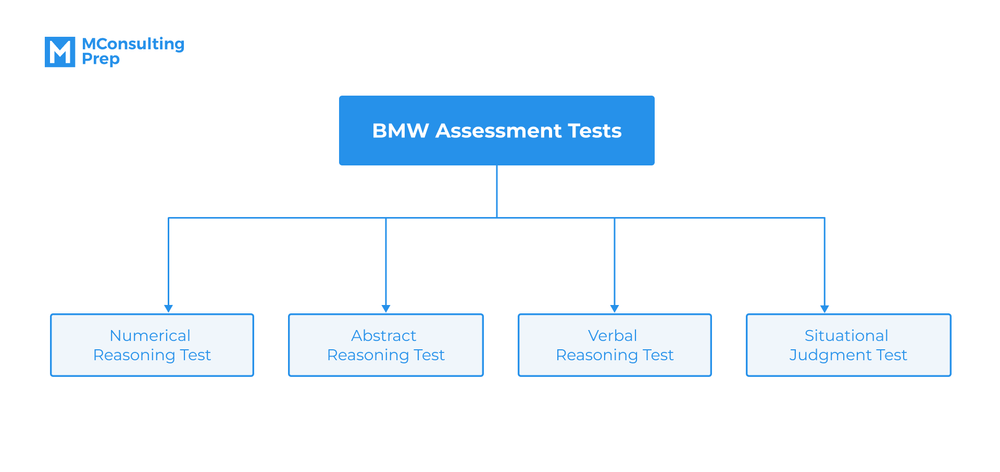• BMW numerical reasoning test: Measure your ability to work with data and solve mathematical problems
• BMW abstract reasoning test: Examine how you understand relationships between shapes and patterns
• BMW verbal reasoning test: Assess your skills in understanding and using written language
• BMW situational judgment test: Find out your work behavior and how you respond to workplace situations
BMW Assessment Tests may also be provided by other test providers in addition to Revelian. Test publishers may have different assessment tests in terms of style and format. However, their ultimate aim is to measure specific skills such as numerical reasoning, verbal reasoning, logical reasoning, etc. Once you master such skills, you may handle any assessment test.

## When do BMW Assessment Tests take place?

BMW Assessment Tests are in the second stage of BMW’s hiring process. If you aim to join BMW, carefully preparing for the online assessment and the whole 4-stage recruitment is crucial. Here is an overview of how BMW selects its job applicants: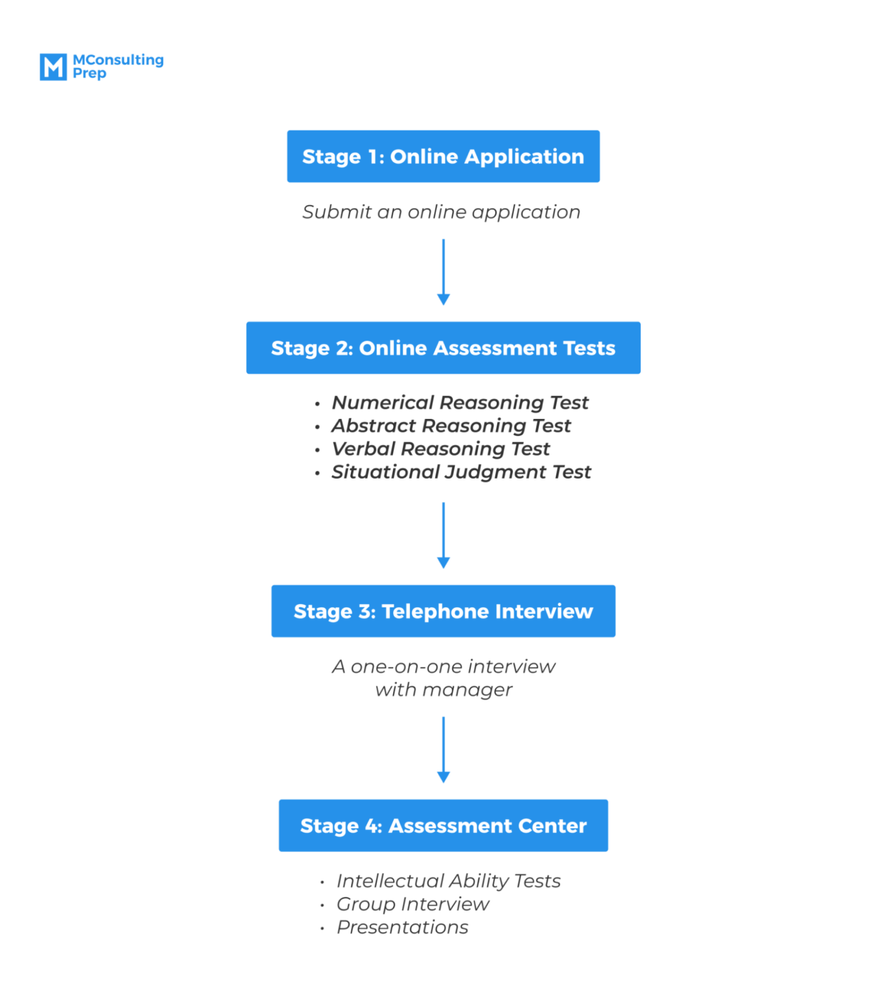Stage 1: Online Application

In this stage, you are required to sign up for the BMW Career Portal, upload your documents and submit an online application. Your application form helps the company gain an overview of your background, personality and motivation, and how these align with BMW’s visions and values.

Stage 2: Online Assessment Tests

After finishing the application form, you will receive an invitation to take BMW Online Tests, which aim to measure candidates’ cognitive abilities. These are tightly timed tests comprising multiple-choice questions in different areas, such as numerical, verbal, logical reasoning, and situational judgment.

Stage 3: Telephone Interview

This is the third stage of BMW’s hiring process, where you will give the company a better understanding of your personality, skills and work experience through a personal interview. Here, not only does BMW ask you, but it is also a chance for you to get all your questions answered.

Stage 4: Assessment Center

This last stage of selection, the so-called Selection Day, includes a series of tasks and activities, including intellectual ability tests, group interviews, or presentations. The assessment center not only gives BMW a deeper insight into its applicants but also helps you to get an overview of how your job at BMW will be.

## BMW numerical reasoning test

In this test, you will show your numerical aptitude by interpreting numerical data, performing arithmetic calculations, and making decisions using numbers. In Revelian-style numerical reasoning tests, there are 3 main question types: Number series, Number matrices, and Number word problems.

### Number series

In this question, you are presented with an arithmetic sequence with one missing number, which you have to determine by finding the pattern that it follows:

Question 1: Replace the question mark with the missing number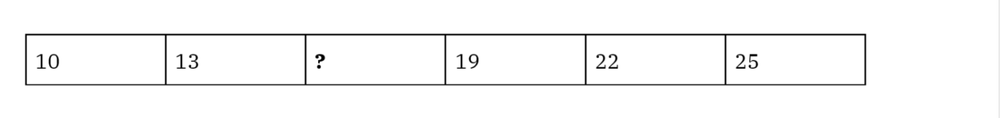Explanation: Each number of the sequence is 3 more than the previous number:

13=10+3

22=19+3

25=22+3

So the answer will be: 13+3 = 16

To solve this test type, you need to find the relation between each pair of consecutive numbers by using basic math calculations: addition, subtraction, multiplication or division. Once noticing the shared pattern in all pairs, you can generate the missing term by making the same calculation to its predecessor or successor.

Let’s look at another question of this type to understand better.

Question 2: Replace the question mark with the missing number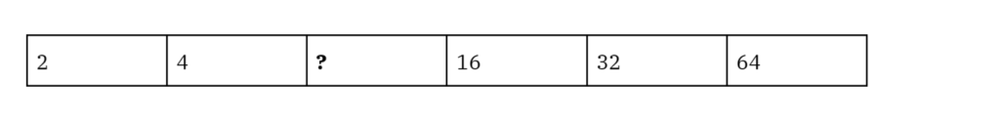Explanation:

The pattern is multiplying the previous term by 2

4=2×2

32=16×2

64=32×2

Therefore, the missing number is: 4×2=8

### Number matrices

This question type shows you a 3×3 number matrix, in which you have to find the missing number presented as a question mark.

Question 3: Find the number that should be in the shaded square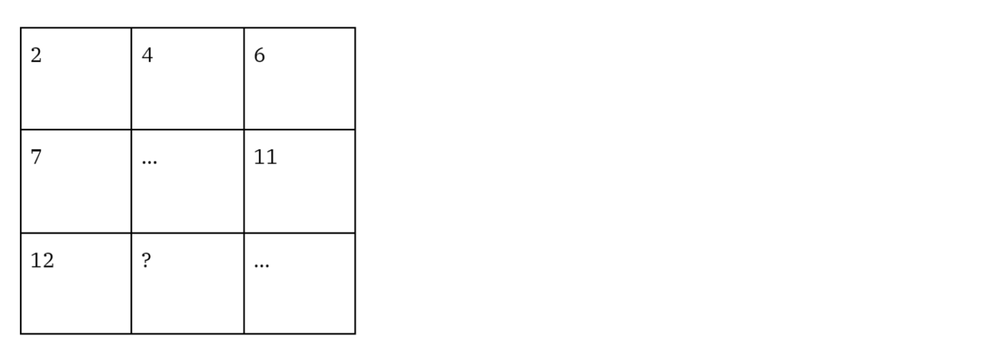Explanation:

Each number in a row is generated by adding 2 to its predecessor:

4=2+2

6=4+2

Meanwhile, the pattern of the column is the addition of 5 to the preceding term:

7= 2+5

12= 7+5

11= 6+5

Therefore, we can find the missing number by adding 2 horizontally or 5 vertically:

12+2 = 14

or

4+5 =9

9+5 =14

So the correct answer is 14.

KEY TAKEAWAYS

In this test type, remember that the 3 rows have the same pattern, and so do the columns. To find the pattern, you can apply the same technique as Number Series; after all, each row or column is a series of numbers.

Let’s practice another example:

Question 4: Find the number that should be in the shaded square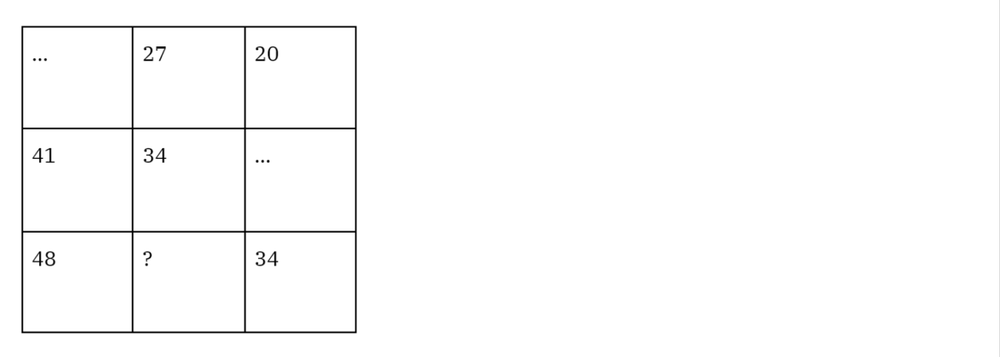Explanation:

In a column, each number ascends by 7:

48=41+7

34=27+7

In a row, each number descends by 7:

20=27-7

34=41-7

Therefore, the missing number will be:

48-7=41

or,

34+7=41

### Number word problems

Number word problems include mathematical questions in written format, involving addition, subtraction, multiplication, and division. The test measures not only your numerical reasoning skills but also your reading comprehension as all the questions are in prose.

Question 5: An oven costs \$80 to produce. The producer made a total profit of \$4 million by selling ovens. If the mark-up percentage is 125%, how many ovens did the producer sell? (Mark-up percentage: The percentage of profits derived over the cost of goods sold).

Explanation:

Mark-up percentage = Profit/Cost x 100%

The cost of all ovens sold is \$4 mil/125% = \$ 3.2 million

The number of ovens sold was 3,200,000/80 = 40,000

So the answer will be 40,000

KEY TAKEAWAYS

Word problems can be challenging since they require you to bridge natural language texts and numerical expressions. However, following this advice can help you ace the test:

• Revise basic calculations and formulas

Numerical reasoning tests are often under a time limit. Therefore, you should know basic mathematical formulas and operations to make quick answers. Word problems are lengthy and confusing, so don’t waste time recalling basic calculations!

• Practice common test types

Several numerical word problems are commonly used: time & work, percentages, ratios & proportion, or profit & loss. Therefore, it is important to practice each test type to determine specific formulas and techniques to solve them.

• Make mathematical notation out of the texts

Texts are hard to follow as they involve reading, so you can convert problems into arithmetic expressions to solve them. Not only math notations but also tables and graphs can be helpful.

## BMW abstract reasoning test

BMW Abstract Reasoning Tests ask you to identify underlying patterns of shapes or symbols. The tests aim to measure your lateral thinking skills or fluid intelligence. Here is a common question type you may encounter regarding the Abstract Reasoning Test:

Question 7: Find the figure in the question mark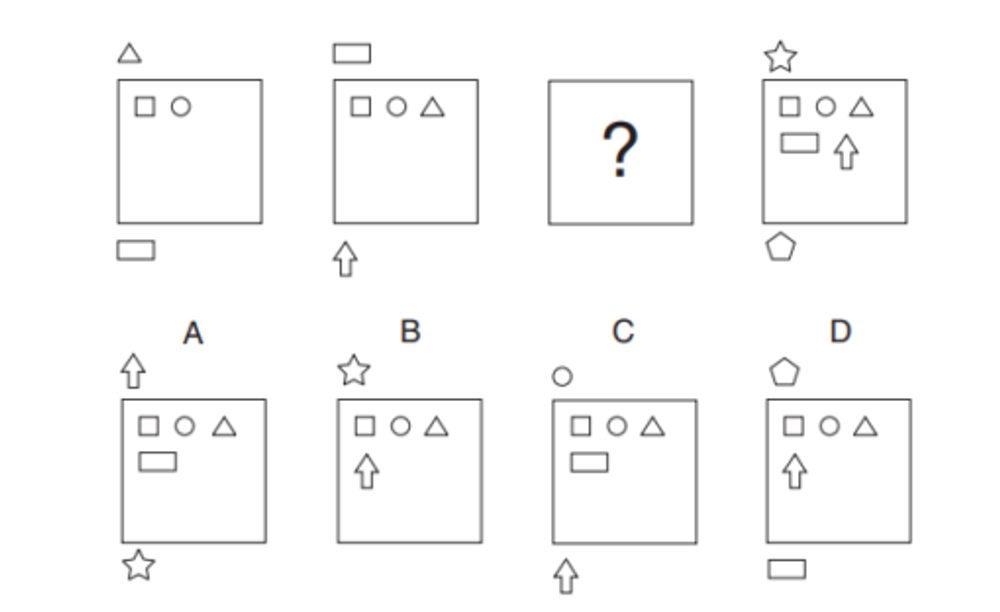Source: How to Pass Diagrammatic Reasoning Tests: Essential Practice for Abstract, Input Type and Spatial Reasoning Tests

Explanation: The top shape from the previous diagram moves into the box at each step, and the bottom shape takes its place.

To pass the Abstract Reasoning Test, there are some details in the figures you need to look for:

• The rotation of figures (both individual or whole)
• The change in colors or positions
• The reflection of shapes and figures
• The increase or decrease in the number of figures

Let’s apply these helpful tips to solve other sample questions below:

Question 8: Which figure is the odd one out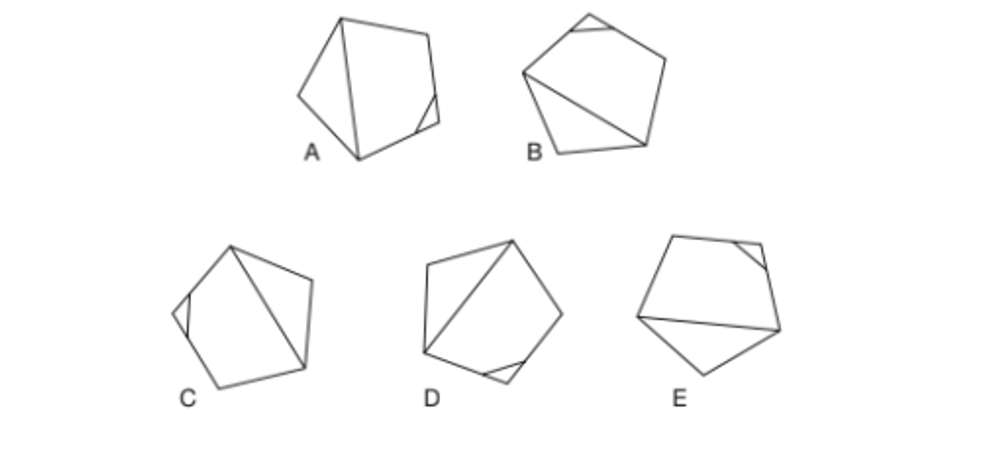Source: The Ultimate IQ Test

Explanation: All the figures, except B, are the same but rotated.

Question 9: Find the missing tile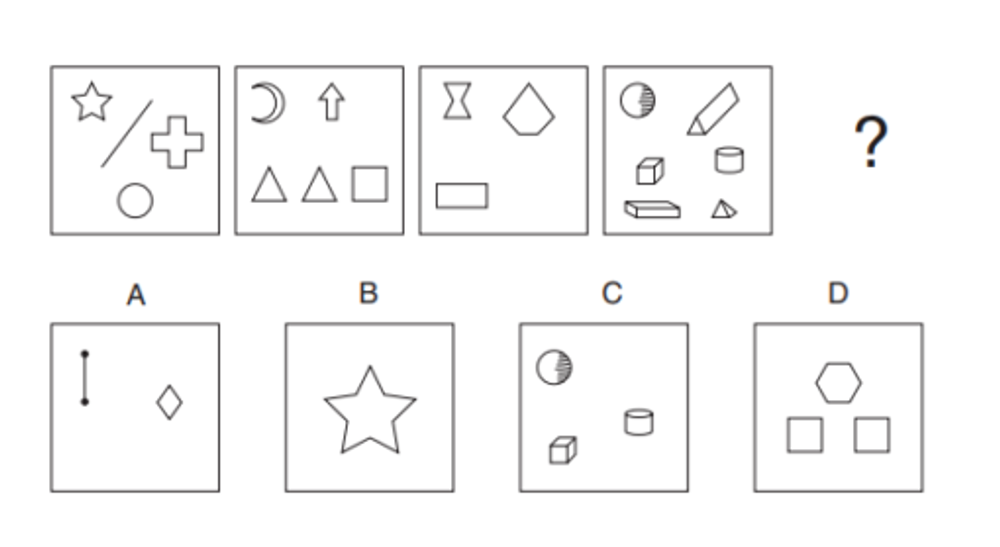Source: How to Pass Diagrammatic Reasoning Tests: Essential Practice for Abstract, Input Type and Spatial Reasoning Tests

Explanation:

Rule 1: The operation alternates between plus and minus

4+1=5

5-2=3

3+3=6

So the number of items in the missing tile will be: 6-4=2

Question 10: Which figure completes the sequence below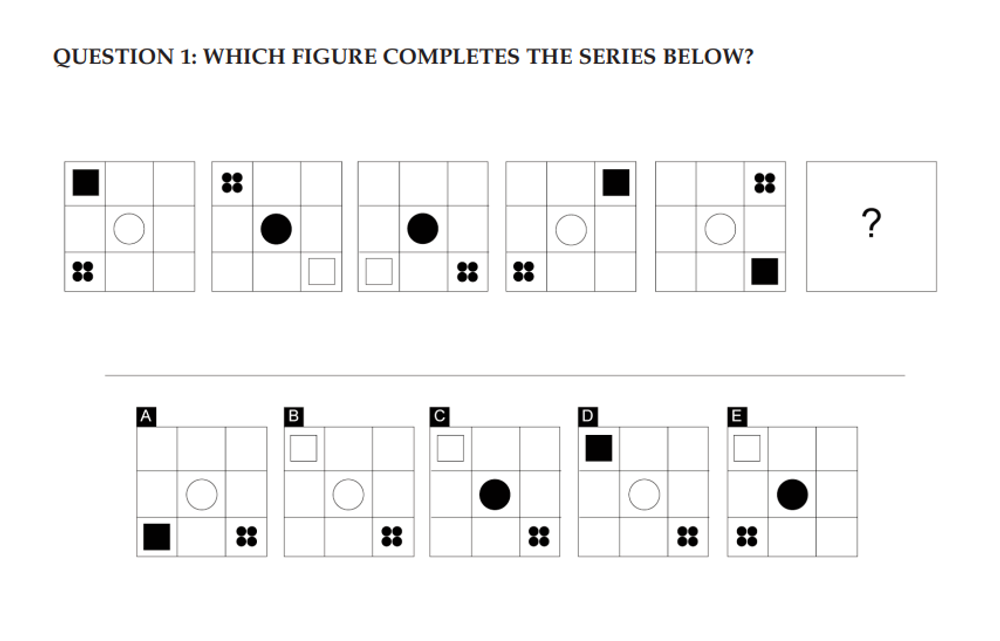Source: The Ultimate EU Test Book

Explanation:

Rule 1: The square’s movement alternates between two steps and one step clockwise between adjacent corners. When it moves two steps, its color changes.

Rule 2: In every other step, the shading of the circle and square are switched.

Rule 3: The group of dots follows the square’s position in the previous step.

## BMW verbal reasoning test

These tests aim to assess your ability to comprehend written information and analyze relationships between words to draw a logical conclusion. BMW Verbal Reasoning Tests consist of 4 main types:

• Synonyms & Antonyms
• Analogy
• Word Associate
• Deductive Reasoning

In Verbal Reasoning Tests, your reading comprehension skills and lexicon are essential qualities that BMW looks for. Hence, a proven tip to ace the test is to increase your extent of vocabulary, practice reading and communicating in different contexts.

### Synonyms & antonyms

This question type gives you two words, and you have to determine whether they are synonyms, antonyms, or neither. This is one of the most widely-used questions in verbal reasoning tests, measuring your range of vocabulary. Here are some examples of this type:

Question 11: Superb – Resplendent. These words…

A. Have similar meanings
B. Have opposite meanings
C. Are neither similar nor opposite

Explanation: Superb means impressively splendid. Resplendent also means attractive and impressive. Therefore, these 2 words are synonyms.

KEY TAKEAWAYS

In this type of questions, you may sometimes encounter some unfamiliar words. In this case, you can look for word root and word form to determine the meaning.

Check out this example.

Question 12: Malicious – Beneficial

A. Have similar meanings
B. Have opposite meanings
C. Are neither similar nor opposite

Explanation:

Malicious means intending to do harm; harmful. Meanwhile, beneficial is favorable or advantageous. Needless to say, they are antonyms.

However, if these words are unfamiliar, you can use word root tips to reach the answer. The prefix ‘mal’ is often used in a negative context, for example, ‘malfunction’ or ‘malnutrition.’ On the contrary, we can easily see that ‘beneficial’ comes from the word ‘benefit,’ indicating a good effect. By that, you can select the answer B though you don’t understand the exact meaning of these words.

### Analogy

Analogy questions test your ability to find the connections between a pair of words. Questions often have two parts; one includes an example of a word pair indicating a specific relationship. Based on the relationship of the example, you have to select the corresponding answer of a given word in the second part.

Question 13: Crime is to Punishment as Earthquake is to …

A. Drought
B. Mining
C. Tectonic
D. Landslide

Explanation: If one commits a crime, they will receive punishment. So this is a cause-effect relationship. ‘Landslide’ is a result from ‘earthquake’, so D would be the correct answer.

KEY TAKEAWAYS

Besides a wide range of vocabulary, you must also identify the exact relationship of the first pair. Maybe you know all the meanings, but if you detect the wrong relationship, you can still fail the test.

Let’s look at this example.

Question 14: Cat is to Mouse as Rabbit is to …

A. Bird
B. Broccoli
C. Carrot
D. Dog

Explanation: All these words are super easy, but the tricky thing is to determine their relationship. ‘Cat’ and ‘mouse’ are all animals, but what is the connection between them? As you know, cats eat mice. So you need to find a food source for a rabbit. Therefore, the correct answer is C, Carrot.

### Word associate

In the Word Associate, you will have to pick one or two words with a different meaning out of the others. In other words, it requires you to understand the semantic relationship between words. For example:

Question 15: Which is the odd one out?

A. Stock
B. Bond
C. Saving
D. Credit Card
E. Insurance

Explanation: Stock, Bond, Saving, and Insurance are types of investments. Meanwhile, ‘Credit Card’ is a mode of payment, which is not related to the others. So, we must choose D for this question.

Question 16: Which two of these words do not belong to the group?

A. Professor
B. Doctor
C. Accountant
D. Bachelor
E. Master
F. Journalist

Explanation: The rest are academic ranks, while ‘Accountant’ and ‘Journalist’ are merely occupations. Thus, C and F are the odd ones out.

### Deductive reasoning

In the BMW Deductive Reasoning Test, you must find 2 premises leading to a given conclusion. This helps BMW assess your deductive logical thinking and problem-solving ability. Now, let’s take a look at how it will be:

Question 17: Which two statements prove that Peter likes Math?

A. Steve likes Math, English, and History
B. All of Steve’s friends like Math
C. Peter is not a friend of Steve
D. Peter likes all the subjects that Steve likes
E. Neither Steve nor Peter is good at Math

Explanation: Steve likes Math, and Peter likes all the subjects that Steve likes. So Peter likes Math as well.

KEY TAKEAWAYS

Remember that the answers are provided within the questions in this test type. Our tip for this test is linking pairs of statements to see the logical flow. Not every 2 statements, just those with relevant information. For example, in the question above, B and C are related to ‘friend’ while A and D are about subject preferences. Thus, you can connect those pairs initially to see if they lead to the given conclusion.

Let’s practice with another example.

Question 18: Which two statements prove that Emily works at MCP Group?

A. Nancy works at MCP Group
B. Nancy and Emily are coworkers
C. MCP Group is 10 kilometers far from Emily’s apartment
D. Emily drives car to work
E. Nancy and Emily live in the same building

Explanation: Nancy works at MCP Group, and she is a colleague of Emily. So, Emily works at MCP Group as well.

## BMW situational judgment test

As well as aptitude tests, BMW uses Revelian Situational Judgment tests to assess candidates’ behavior, personality, and working style. The tests often include a statement or a scenario followed by multiple-choice answers. By that, BMW can determine whether a job applicant will be a good fit for the company.

To ace the Situational Judgment Test, you have to keep in mind these guidelines:

• Understand the employer and the role you apply for. BMW uses this test to find consistent candidates. Thus, if you know what BMW is looking for and highlight those qualities in your answer, it is likely that the company will see you as a good fit.
• Read the scenario and each response carefully. Sometimes, you immediately see a good response for a situation, but maybe there’s a more sensible one among the rest. Therefore, take your time and read all the answers carefully, and compare one with another if necessary.